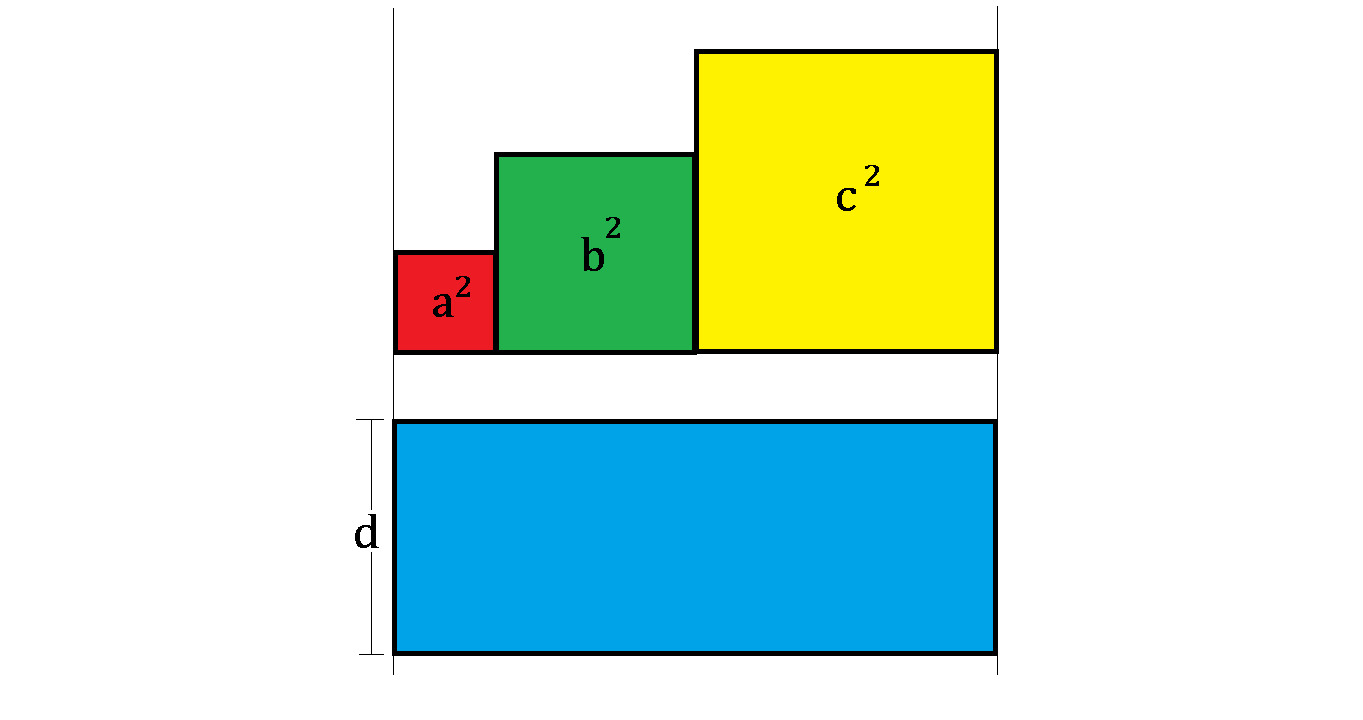Width MysteryAs shown above, the 3 colored squares have the side lengths of $a while the blue rectangle has the length of $a+b+c$ and the width of $d$.

If the blue rectangle's area is equal to the sum of 3 squares' areas combined, which of the following statements is correct?

×